# 数据结构与算法(8)-排序(冒泡 插入 选择)

• 这里介绍三大类型的排序
• 冒泡，插入，选择 O(n^2)
• 快排，归并 O(nlogn)
• 桶，计数，基数

# 1. 如何分析一个排序算法？

## 1.1 执行效率

• 最好情况，最坏情况，平均情况时间复杂度
• 要知道针对于数据集的特征，需要采取哪一种排序算法
• 时间复杂度的系数，常数，低阶
• 实际开发过程中，面对规模比较小的数据，我们可能需要将系数，常数，低阶都考虑进去才可以的
• 比较次数和交换次数
• 对于基于比较的排序算法，会涉及到元素大小的比较以及元素的交换或者移动，因此当我们在分析排序算法的执行效率的时候，应该把比较次数和交换次数也考虑进去

# 2. 算法分析

## 2.1 冒泡排序

• 操作相邻的两个数据
• 每次冒泡都会对相邻的两个元素进行比较，
• 看是否满足大小关系要求
• 如果不满足就让它倆互换，一次冒泡至少会让一个元素移动到它应该在的位置，重复n次，就完成了n个数据的排序工作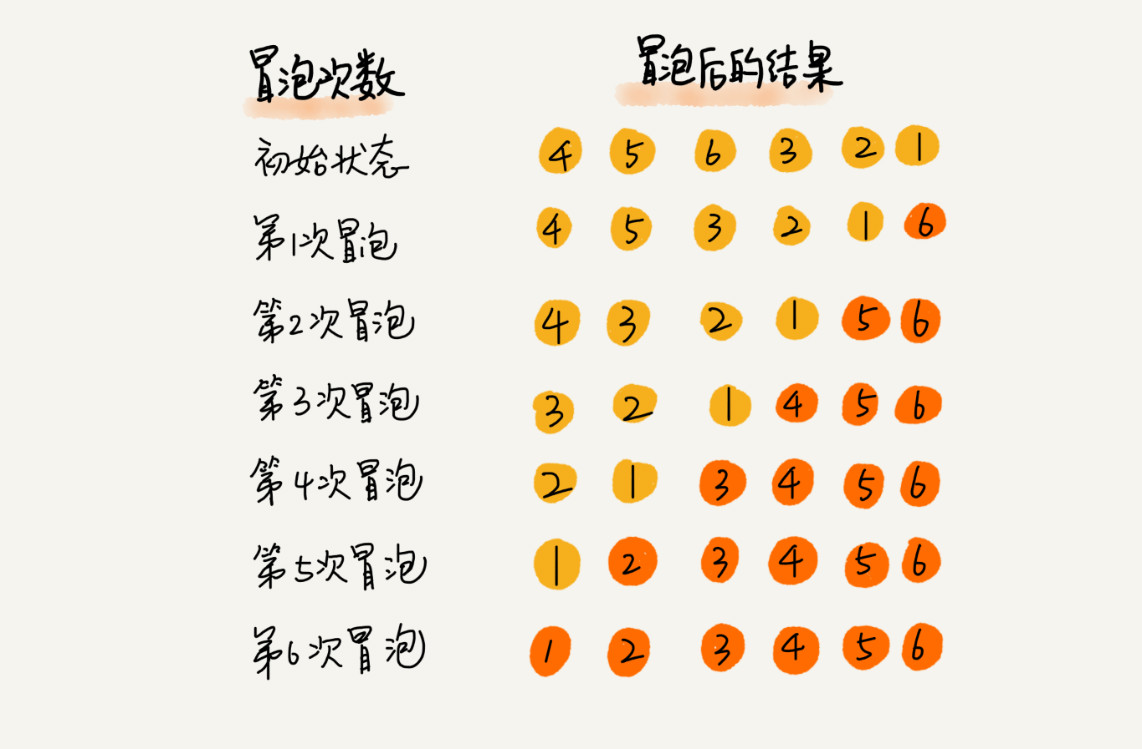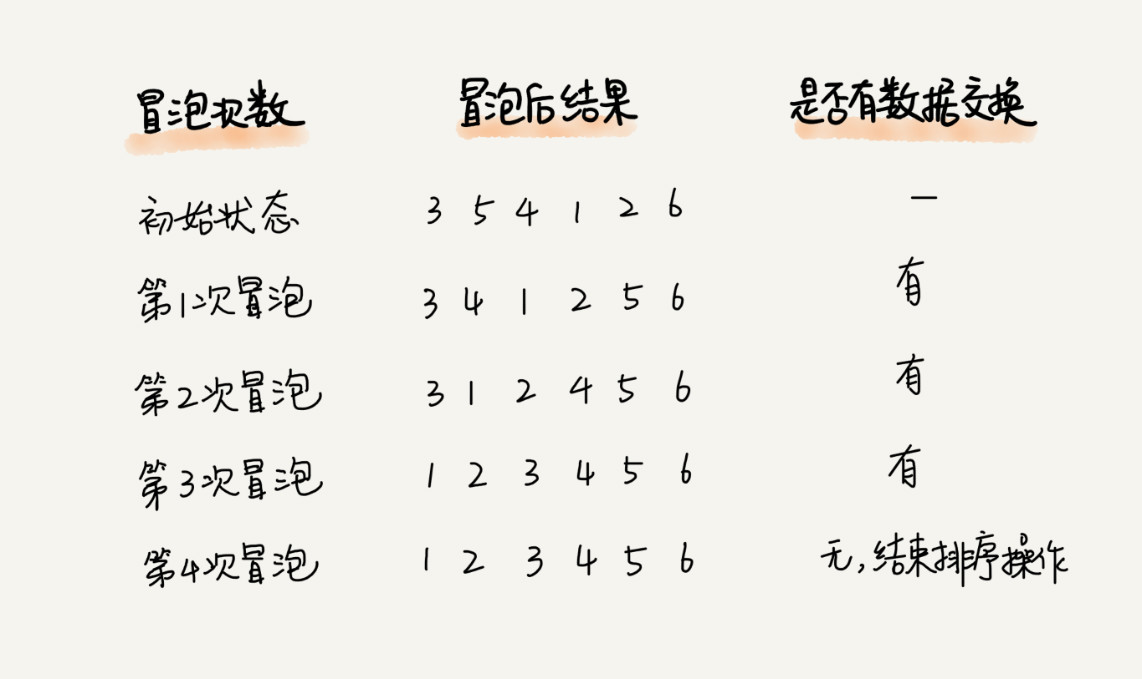``````// 冒泡排序，a 表示数组，n 表示数组大小
public void bubbleSort(int[] a, int n) {
if (n <= 1) return;

for (int i = 0; i < n; ++i) {
// 提前退出冒泡循环的标志位，如果没有冒泡，说明已经有序了，不用再进行下去了
boolean flag = false;
// -i是因为在进行第i轮次的时候，最末尾的是已经排好了的i个数
for (int j = 0; j < n - i - 1; ++j) {
if (a[j] > a[j+1]) { // 交换
int tmp = a[j];
a[j] = a[j+1];
a[j+1] = tmp;

flag = true;  // 表示有数据交换
}
}
if (!flag) break;  // 没有数据交换，提前退出
}
}``````
• 冒泡排序是原地排序算法，空间复杂度为O(1)
• 冒泡排序是稳定的排序算法
• 时间复杂度
• 最好情况 O(n)
• 最坏情况 O(n^2)

## 2.2 插入排序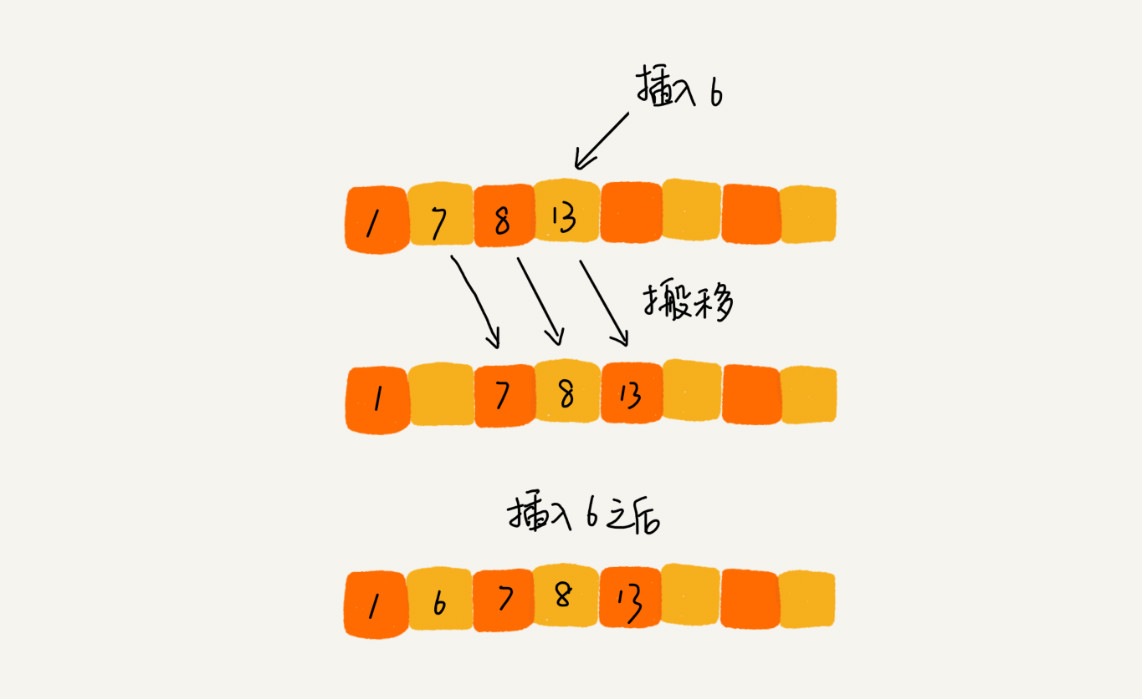• 将数组中的数据分为两个区间，已排序空间和未排序空间
• 初始已排序空间有一个元素，即数组的第一个元素
• 插入算法的核心思想是取未排序区间当中的元素，在已排序空间中找到合适的插入位置将其插入，并保证已排序空间的数据一致都是有序的
• 重复这个过程直到未排序空间中的元素为空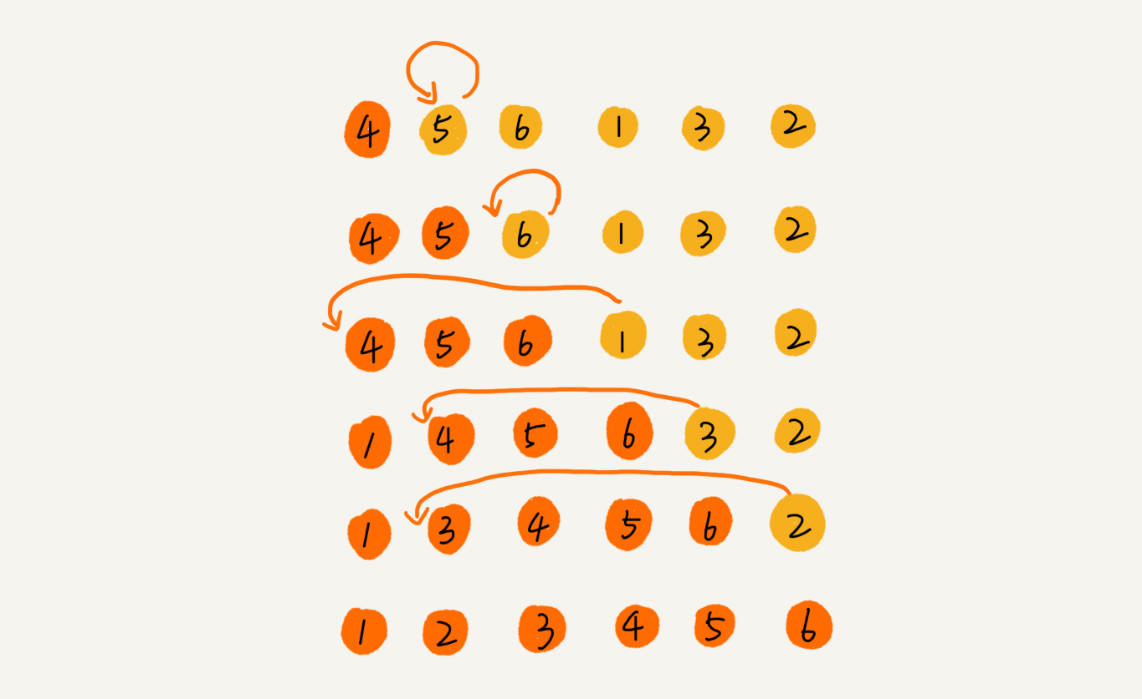``````// 插入排序，a 表示数组，n 表示数组大小
public void insertionSort(int[] a, int n) {
if (n <= 1) return;

for (int i = 1; i < n; ++i) {
int value = a[i];
int j = i - 1;
// 查找插入的位置
for (; j >= 0; --j) {
if (a[j] > value) {
a[j+1] = a[j];  // 数据移动
} else {
break;
}
}
// 这里注意循环当中j--了，所以这里是a[j+1]
a[j+1] = value; // 插入数据
}
}``````
• 原地排序算法，空间复杂度为O(1)
• 稳定的排序算法
• 时间复杂度
• 最好情况O(n)
• 最坏情况O(n^2)

## 2.3 选择排序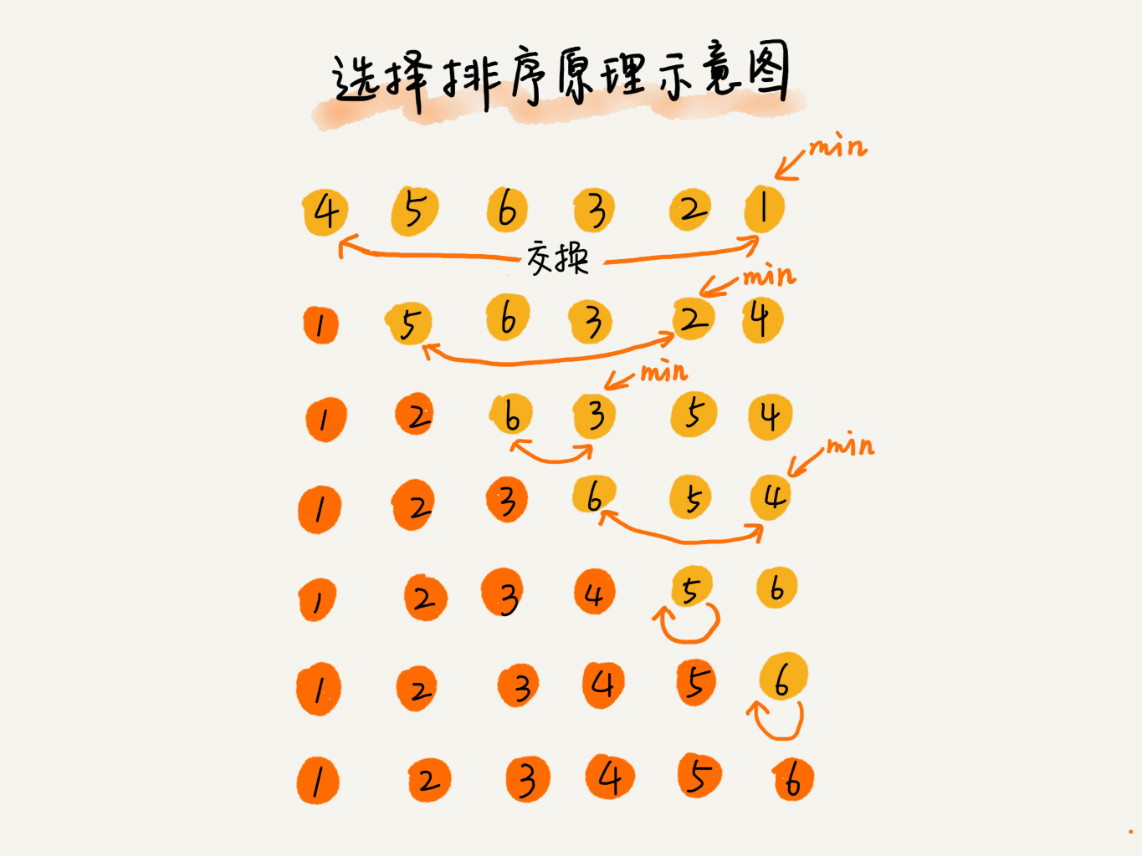• 原地排序算法

• 不稳定的，因为每次都要找剩余的未排序的元素当中的最小值，并和前面的元素交换位置

public int[] selectSort(int arr[], int n) {

``````for (int i = 0; i < n; i++) {
int index = min(i+1, n);
swap(arr[index], arr[i]);
}``````
``````  return arr;
}``````

# 3. 冒泡 插入 选择排序的比较

×